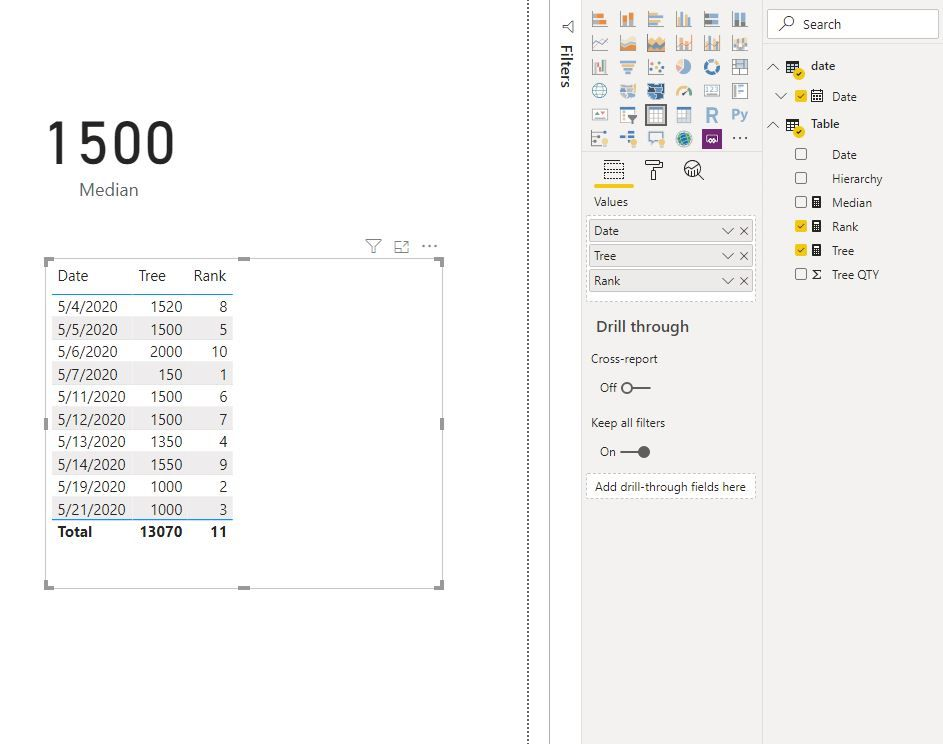cancel
Showing results for
Did you mean:Helper I

## exclude blanks from median for measure

Hi i'm trying to get the median of a measure and exclude the blank values. I have a table below. The result I'm trying to get is 1500 but my measure keeps coming up blank.

The "Trees" column is a measure, see below.

The date is taken from my Dim Date table.

Measure for "Trees" : CALCULATE(DISTINCTCOUNT('Trees'[Tree QTY]),'Trees'[Hierarchy]="Hacyinth")

 Date Trees 5/6/2020 2000 5/21/2020 1000 5/19/2020 1000 5/5/2020 1500 5/12/2020 1500 5/11/2020 1500 5/14/2020 1550 5/4/2020 1520 5/13/2020 1350 5/7/2020 150 5/18/2020 5/20/2020 5/25/2020 5/26/2020 Median 1500

Can someone help? Seems like it should be easy but it is not working with MEDIANX

The formula I have is

Median =
MEDIANX('Trees', [Tree Qty])
1 ACCEPTED SOLUTIONHelper I

Thanks everyone, I Found another thread that provided a soultion that worked: https://community.powerbi.com/t5/Desktop/Median-of-a-column-in-Table-Visualization/td-p/582142

```=
MEDIANX (
SUMMARIZE ( Data, Data[Iteration] ),
"Total Points", 1.0 * CALCULATE ( SUM ( Data[Points] ) )
),
[Total Points]
)```
9 REPLIES 9Community Support

For the dates which has blank value of "Trees", are they in "Tree" table for 'Trees'[Hierarchy]="Hacyinth"?

If all blank values are regarded as one, for example, your example above has 11 numbers for calculating median.

blank 150 1000 1000 1350 1500 1500 1500 1520 1550 2000

If so, try workarounds below:``````Rank =
IF (
[Tree]
<> BLANK (),
RANKX (
FILTER (
ALL ( 'date'[Date] ),
[Tree]
<> BLANK ()
),
RANKX (
FILTER (
ALL ( 'date'[Date] ),
[Tree]
<> BLANK ()
),
[Tree],
,
ASC,
DENSE
)
+ DIVIDE (
RANKX (
FILTER (
ALL ( 'date'[Date] ),
[Tree]
<> BLANK ()
),
CALCULATE (
MAX ( 'date'[Date] )
),
,
ASC,
DENSE
),
(
COUNTROWS (
FILTER (
ALL ( 'date'[Date] ),
[Tree]
<> BLANK ()
)
) + 1
)
),
,
ASC,
DENSE
)
)

Median =
VAR max1 =
MAXX (
ALL ( 'date' ),
[Rank]
)
VAR mo =
MOD (
max1 + 1,
2
)
RETURN
SWITCH (
TRUE (),
mo = 1, CALCULATE (
[Tree],
FILTER (
'date',
[Rank] = max1 / 2
)
),
mo = 0, DIVIDE (
CALCULATE (
[Tree],
FILTER (
'date',
[Rank] = ( max1 - 1 ) / 2
)
)
+ CALCULATE (
[Tree],
FILTER (
'date',
[Rank] = ( max1 + 1 ) / 2
)
),
2
)
)

``````

Best Regards
Maggie
Community Support Team _ Maggie Li
If this post helps, then please consider Accept it as the solution to help the other members find it more quickly.Helper I

Thanks everyone, I Found another thread that provided a soultion that worked: https://community.powerbi.com/t5/Desktop/Median-of-a-column-in-Table-Visualization/td-p/582142

```=
MEDIANX (
SUMMARIZE ( Data, Data[Iteration] ),
"Total Points", 1.0 * CALCULATE ( SUM ( Data[Points] ) )
),
[Total Points]
)```Community Champion

Hi @RyndaRaw ,

I think you must use the function MEDIAN(). It doesn't include blank values.

Regards FrankATHelper I

I can't use MEDIAN() with a measure though.Community Champion

if [Trees] is your measure as you mentioned before, you can create a measure =

``MEDIANX(Table, [Trees])``

do not hesitate to give a kudo to useful posts and mark solutions as solutionHelper I

MedianX(table,[measure]) doesn't work, I keep getting a blank valueCommunity Champion
``CALCULATE(MEDIANX(Table, [Trees]), ALL(Table) )``

do not hesitate to give a kudo to useful posts and mark solutions as solutionHelper I

THat gives me the median of all the values in the table. It doesn't aggregate the values by date first and then take the median of those aggregated values.

😕Microsoft

Median Measure = MEDIANX(VALUES('Trees'[Date]), [Trees])

If this works for you, please mark it as solution.  Kudos are appreciated too.  Please let me know if not.

Regards,

Pat

Did I answer your question? Mark my post as a solution! Kudos are also appreciated!